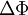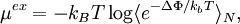# Difference between revisions of "Widom test-particle method"This article is a 'stub' page, it has no, or next to no, content. It is here at the moment to help form part of the structure of SklogWiki. If you add sufficient material to this article then please remove the {{Stub-general}} template from this page.
the excess chemical potential of a system that is being simulated. A test particle is introduced in a random location, and$\Delta\Phi$, the difference in internal energy before and after the insertion, is computed. (For pairwise interactions, this would just be the interaction potential energy between the randomly placed test particle and the N particles that the system is comprised of.) The particle is not actually inserted (at variance with grand canonical MC ).$\mu^{ex} = -k_BT \log \langle e^{-\Delta\Phi/k_bT}\rangle_N ,$
where$k_B$ is the Boltzmann constant and T is the temperature.